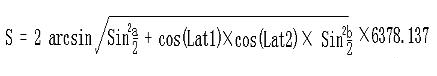# 使用 MySQL 实现搜索附近的人

10 May 2017

## 背景介绍

``````商店: 找到你当前的位置;

``````

## 经纬度

### 距离计算

``````C = sin(MLatA)*sin(MLatB)*cos(MLonA-MLonB) + cos(MLatA)*cos(MLatB)
Distance = R*Arccos(C)*Pi/180

``````

google 地图提供的方法则是如下公式:## 实现方式

### redis

redis 从 3.2 版本开始支持位置信息的操作, 提供了 6 个相关的操作方法分别为以下:

``````GEOADD:  向指定的 key 中增加位置成员;
GEODIST: 计算指定 key 中两个成员之间的距离;
GEOHASH: 返回指定成员的 geohash 结果;
GEOPOS:  返回指定 key 中成员的位置信息;
``````

``````优点:
1. 简单, redis 接口调用即可, 方法也不多;
2. 适合集合较少的应用;

1. 集合太大容易引起性能瓶颈;
2. 没有过期时间和删除成员的方法,  需要开发人员根据业务规则遍历 key 清除;
3. 没有额外的 hook 相关的功能, 需要开发人员做很多工作;
``````

### tile38

tile38 是新兴且开源的地理位置数据库, 兼容 redis 协议. 看活跃程度国外还有人用, 国内就没怎么发现. 如果选用 tile38 则需要考虑一下优缺点:

``````优点:
1. 功能函数丰富, 有很多惊喜的功能, 比如超时设置, hook 调用通知, json 接口等;
2. golang 语言编写, 部署方便;
3. 支持主从, 以 redis 的 aof 格式存储数据;
4. 搜索函数比起 redis 丰富了很多;

1. 不是特别稳定, github 还有很多未解决的 issue;
2. 没有配置文件, 都在代码里控制, 不清楚作者有没有这方面的打算;
3. 内存使用和磁盘刷新的策略还不明朗, 需要读代码才能确定;
``````

### Mongodb

Mongodb 也提供了地理位置相关的功能, 操作函数较丰富, 几何方面的支持也很全, 不过不能使用地理位置索引作为分区的键. 读者可以参考使用 mongodb 完成地理位置相关的功能;

### Postgis

PostgreSQL 的扩展 Postgis 则提供了很全面的空间相关的操作函数, 基于 OpenGIS 实现了很多几何空间相关的函数, 同样 postgis 也支持空间相关的索引. 国内使用 postgresql 的人不多, 如果要选用该扩展, 学习成本较大, 另外成员的过期时间需要开发者实现.

### MySQL

MySQL 从 5.6 开始基于 OpenGIS 实现了很多空间相关的函数, 不过仅 MyISAM 引擎支持空间索引, 在 5.7 中 InnoDB 引擎才开始支持空间索引. 功能上类似 PostGIS, 也提供了很多几何空间方面的操作函数, 比如点, 线, 多边形的操作等, 不过其存在时间不长, 比起 postgis 功能上也不是特别丰富, 一些位置距离的计算需要靠开发者自己完成, 相关的过期时间也需要开发者完成. 详细可参考文章 mysql-spatial-functions , 下文主要介绍以传统的方式计算两个地理位置之间的距离, MySQL 的空间数据类型可参考 Spatial Data Type, 下文会给出一个简单的实例.

## MySQL 计算位置之间的距离

``````表结构:
CREATE TABLE `geotest` (
`userid` int(10) NOT NULL,
`longitude` decimal(9,6) NOT NULL,
`latitude` decimal(9,6) NOT NULL,
`create_time` datetime DEFAULT NULL,
UNIQUE KEY `unq_uid` (`userid`),
KEY `idx_lat_lng` (`longitude`,`latitude`)
) ENGINE=InnoDB DEFAULT CHARSET=utf8

insert geotest values(10000, 116.417480, 40.003033, now());
insert geotest values(10001, 116.437480, 40.004033, now());
insert geotest values(10002, 116.457480, 40.005033, now());
insert geotest values(10003, 116.477480, 40.006033, now());
......
......

``````

``````C = sin(MLatA)*sin(MLatB)*cos(MLonA-MLonB) + cos(MLatA)*cos(MLatB)
Distance = R*Arccos(C)*Pi/180
``````

``````R*arccos( cos( radians(latA)*cos( radians(latB) ) * cos( radians(lonA - lonB) )) + sin( radians(latA)*cos(latB) ))
``````

``````SELECT
user_id, (
6371 * acos (
* cos( radians( latitude ) )
* sin( radians( latitude ) )
)
) AS distance
FROM geotest
HAVING distance < 1
ORDER BY distance
LIMIT 0 , 20;
``````

``````1°latitude  ~= 69 miles
1°longitude ~= cos(latitude)*69 miles
``````

``````  +-------------+
|             |
|             |
|      +      |
|             |
|             |
+-------------+
``````

``````lng1 = lon - dist/abs(cos(radians(lat))*69)
lat1 = lat - (dist/69);
lat2 = lat + (dist/69);
``````

``````SELECT
user_id, (
6371 * acos (
* cos( radians( latitude ) )
* sin( radians( latitude ) )
)
) AS distance
FROM geotest
WHERE longitude BETWEEN lng1 AND lng2
AND latitude BETWEEN lat1 AND lat2
HAVING distance < 1
ORDER BY distance
LIMIT 0 , 20;
``````

``````DELIMITER \$\$
drop procedure if exists geo_dist\$\$
create procedure geo_dist(IN lng decimal(9, 6), IN lat decimal(9, 6), IN dist int)
begin
declare lng1 decimal(9, 6); declare lng2 decimal(16, 13);
declare lat1 decimal(9, 6); declare lat1 decimal(16, 13);

-- calculate lng and lat for the rectangle, in meters unit
set lng1 = lng - dist/abs(cos(radians(lat))*111044.736);
set lng2 = lng + dist/abs(cos(radians(lat))*111044.736);
set lat1 = lat - (dist/111044.736);
set lat2 = lat + (dist/111044.736);

-- run the query
select user_id, round((
6371392.89 * acos (
* cos( radians( latitude ) )
* sin( radians( latitude ) )
)
), 0) AS distance
from user_position
where lng between lng1 and lng2
and lat between lat1 and lat2
having distance < dist
ORDER BY distance
LIMIT 0 , 20;
END\$\$
DELIMITER ;
``````

``````mysql > call geo_dist(116.4174800000000, 40.0030330000000, 5000);
+---------+----------+
| user_id | distance |
+---------+----------+
|   10000 |        0 |
|   10001 |     1707 |
|   10002 |     3414 |
+---------+----------+
3 rows in set (0.00 sec)

Query OK, 0 rows affected (0.01 sec)
``````

10001 用户和指定的经纬度距离为1707米, 我们在 redis 3.2 版本中进行简单测试, 可以看到结果都很相近:

``````127.0.0.1:6380> geoadd tttt 116.417480 40.003033 t1
(integer) 0
127.0.0.1:6380> geoadd tttt 116.437481 40.004034 t2
(integer) 0
127.0.0.1:6380> GEODIST tttt t1 t2
"1707.5093"
``````

``````CREATE TABLE `geotest` (
`userid` int(10) NOT NULL,
`create_time` datetime DEFAULT NULL,
UNIQUE KEY `unq_uid` (`userid`),
) ENGINE=InnoDB DEFAULT CHARSET=utf8

mysql > insert geotest values(10000, POINT(116.417480  40.003033), now());
mysql > insert geotest values(10001, POINT(116.437480, 40.004033), now());
mysql > insert geotest values(10002, POINT(116.457480, 40.005033), now());
mysql > insert geotest values(10003, POINT(116.477480, 40.006033), now());

mysql > select userid, ST_Distance_Sphere(POINT(116.4174800000000, 40.0030330000000), geoaddr) as distance
from geotest
where ST_Distance_Sphere(POINT(116.4174800000000, 40.0030330000000), geoaddr) < 5000;
+--------+--------------------+
| userid | distance           |
+--------+--------------------+
|  10000 |                  0 |
|  10001 | 1707.1384059048478 |
|  10002 | 3414.2518917034054 |
+--------+--------------------+
3 rows in set (0.00 sec)

mysql > set @pt1 = ST_GeomFromText('POINT(116.417480 40.003033)');
Query OK, 0 rows affected (0.00 sec)

mysql > set @pt2 = ST_GeomFromText('POINT(116.437481 40.004034)');
Query OK, 0 rows affected (0.00 sec)

mysql > select ST_Distance_Sphere(@pt1, @pt2) as distance;
+--------------------+
| distance           |
+--------------------+
| 1707.2306341756278 |
+--------------------+
1 row in set (0.01 sec)
``````